## Programming and Anna Weltman’s This Is Not A Math Book and Dan Meyer’s Contest

PSA: check out Anna Weltman’s new book, This Is Not A Math Book. Looks great.
Also check out Dan Meyer’s fall contest (ends 10/6/15) that asks the students to be creative in making their own Loop-de-loop mathematical art.
Programming connection: have the students make their loop-de-loop with python and turtles, here is a 3-5-2 loop-de-loop: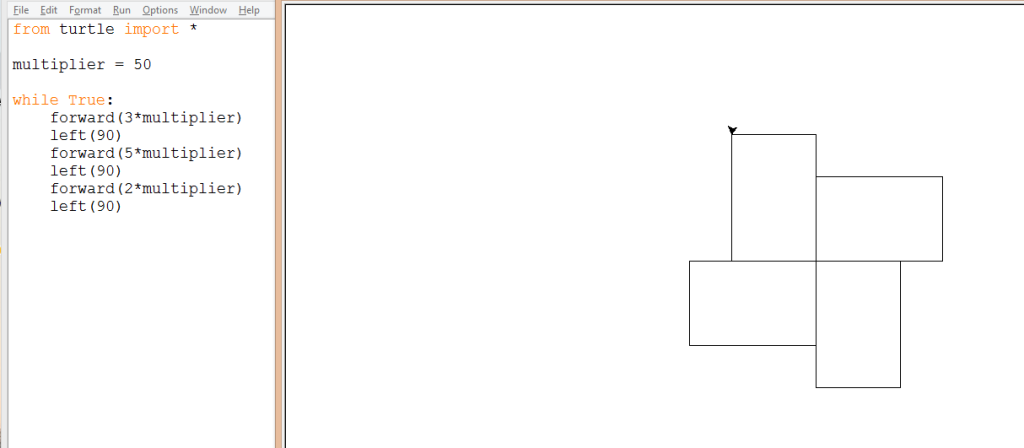or with Scratch, here is a 2-4-5:Enjoy!

Posted in interesting stuff | Tagged , | 2 Comments

## BetterQs Blog

At TMC 2015 I attended a fantastic session by Rachel Kernodle that encouraged us to improve our questioning in class. I took this goal to heart, in fact, it ended up being my “One TMC15 Thing” that I’d like to work on.

Thankfully this message found much traction in the #mtbos. Rachel and Sam Shah started up the BetterQs blog where many guests have written posts on questioning. I wrote a post today, Hints and One Helpful Question. If you’d like, go over and read it, and considering adding BetterQs to your blog aggregator of preference.
Thanks,
Dan## Car Talk: False Positive?

RAY: There’s a rare disease that’s sweeping through your town. Of all the people who are exposed to it, 0.1 percent of the people actually contract the disease. There are no symptoms until the disease actually occurs. However, there’s a diagnostic test that can detect the presence of the disease up to a year before it strikes. You go to your doctor, and he administers the test. It comes out positive. You say, “I’m done for!” Then you get a little bit encouraged. You say, “Wait a minute, doc, is this test 100 percent accurate?” Your doctor responds, “Well, not really. It’s 95 percent accurate.” In other words, 5 percent of the people who take the test will test positive but they don’t really have the disease. Here’s the question: What are the chances that you actually have the disease?

Posted in Uncategorized | 4 Comments

## Car Talk: Barber Math

From the fantastic car talk puzzler:

NEW BARBER MATH
RAY: A barber had his first customer of the day, who happened to be a friend. When he was done, the barber refused to take the money from the customer. The fellow said, “Look, I know we’re friends, but, business is business. I want to pay for my haircut.”

The barber said, “Here’s what we’ll do. You open the cash register. I don’t have any idea how much money is in there. But, you match whatever is in there, and then take out 20 bucks.”

The customer says, “Okay,” and he does that.

The barber says, “Gee, I kind of like this.” So, the next customer comes in, he gets his haircut, and the barber says, “You can do the same thing my first customer did. Open the cash register, match what’s in there, and then take out 20 bucks.”

The second customer does that, and he leaves. The third customer does the same. The fourth customer, after receiving his haircut, opens the cash register, and says, “I can’t do it. ”

The barber says, “Why not?”

“There’s no money in here. Not a cent.”

The question is, how much money was in there to start?

Posted in interesting stuff | Tagged , | 3 Comments

## Plots of the Exponential Function over the Complex Plane

I was inspired by this tumblr post, and this wikimedia page to make some pretty pictures of the exponential function over the complex plane. Go there to read in more detail how the images were created, including how the colors were created.
Code is found here.
Hi definition images are found here.Update: Here’s what happens if you zoom in on one of these graphs.Posted in Full Posts | Tagged , | Leave a comment

## Tracking Venus and Earth over 8 years

It reminded me strongly of the waning moon and linear mod art projects that I’ve been playing with.

For instance, here’s a screenshot from the waning moon (specifically y = 56x)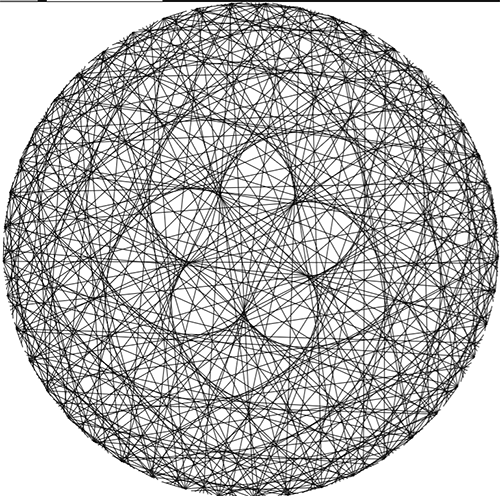A quick intro: the picture above was made by taking 360 points around a circle, and shifting them by the function (y = 56x), and then graphing a line between the input and output. If the output is greater than 360, then take the remainder after dividing by 360.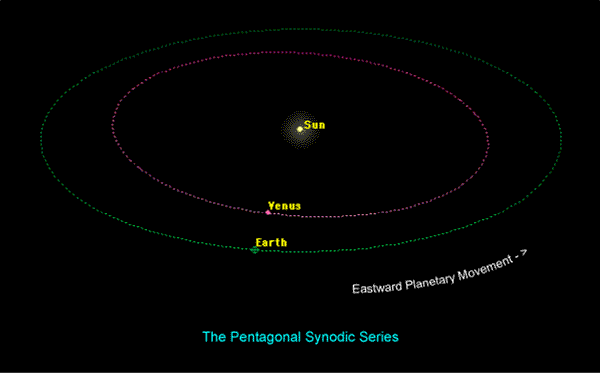From lunar planner.

And from John Carlos Baez’s blog:

It’s called the pentagram of Venus, because it has 5 ‘lobes’ where Venus makes its closest approach to Earth. At each closest approach, Venus move backwards compared to its usual motion across the sky: this is called retrograde motion.

Actually, what I just said is only approximately true. The Earth orbits the Sun once every

365.256

days. Venus orbits the Sun once every

224.701

days. So, Venus orbits the Sun in

224.701 / 365.256 ≈ 0.615187

Earth years. And here’s the cool coincidence:

8/13 ≈ 0.615385

That’s pretty close! So in 8 Earth years, Venus goes around the Sun almost 13 times. Actually, it goes around 13.004 times.

Anyway, there’s a lot to investigate here. How is the function y = 56x related to these planetary orbits?

Posted in Full Posts | | 4 Comments

## Linear Modulus Art

I saw this fantastic webpage a couple of months back.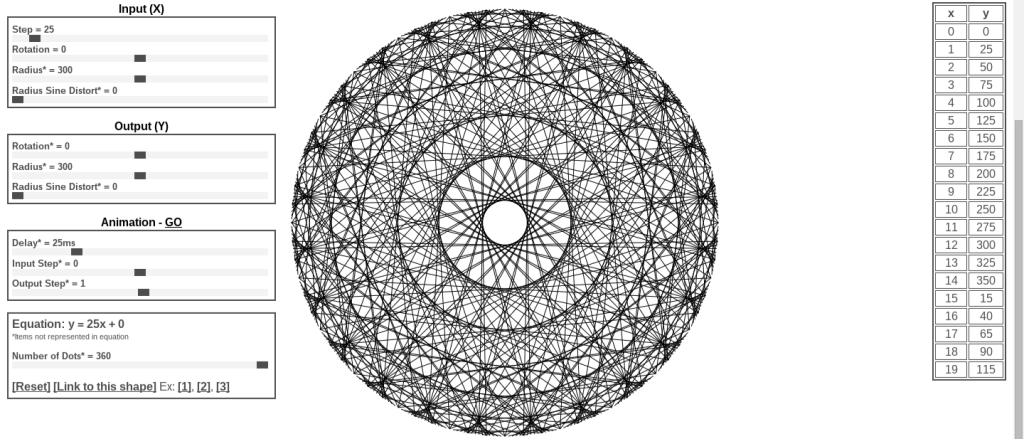Tons of fun. I took that idea and made a gif for my weird art Tumblr, Recursive Processing.Here’s the processing.org code and the live (and smoother) version, and here’s the more fun version that is interactive based on the mouse position (some interesting polar math is involved here, x = r cos (theta) and y = r sin (theta) ).

These all work on the same principle, take a bunch of points around a circle, and shift them around the circle based on a linear function. If the output is too large, then they wrap around (clock math). The formal name for this idea is modular arithmetic, a very useful concept in computer programming. A quick example: Take an input value of x = 120, and a function of y = 3x + 20 (mod 360). y = 3 (120) + 20 (mod 360) = 380 (mod 360) = 20 (the remainder after dividing by 360). So make a line between 120 on the circle and 20. The resulting image after calculating 360 different points for y = 3x + 20 is: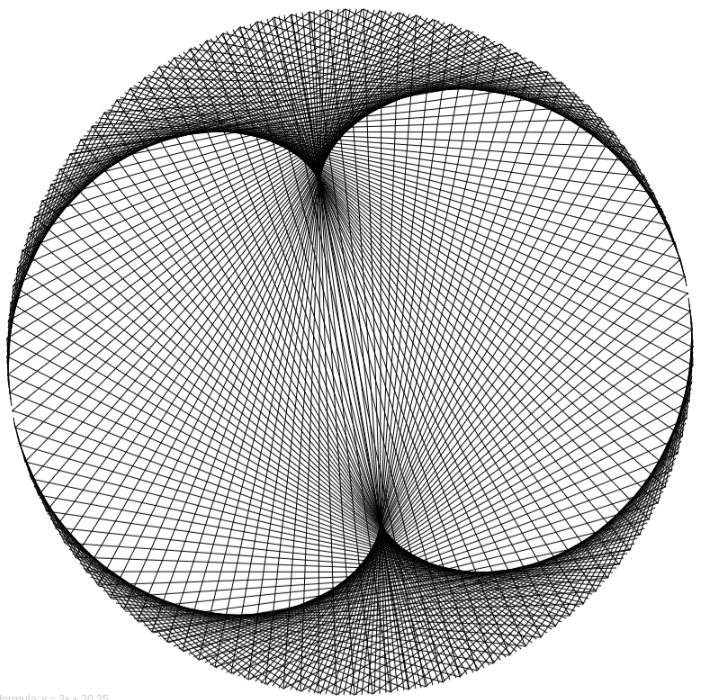But this concept is more difficult to grasp with the unusual step of graphing the points around a circle.

## Separate Input from Output

Lets go to a more straightforward representation. Here’s an interactive that might help.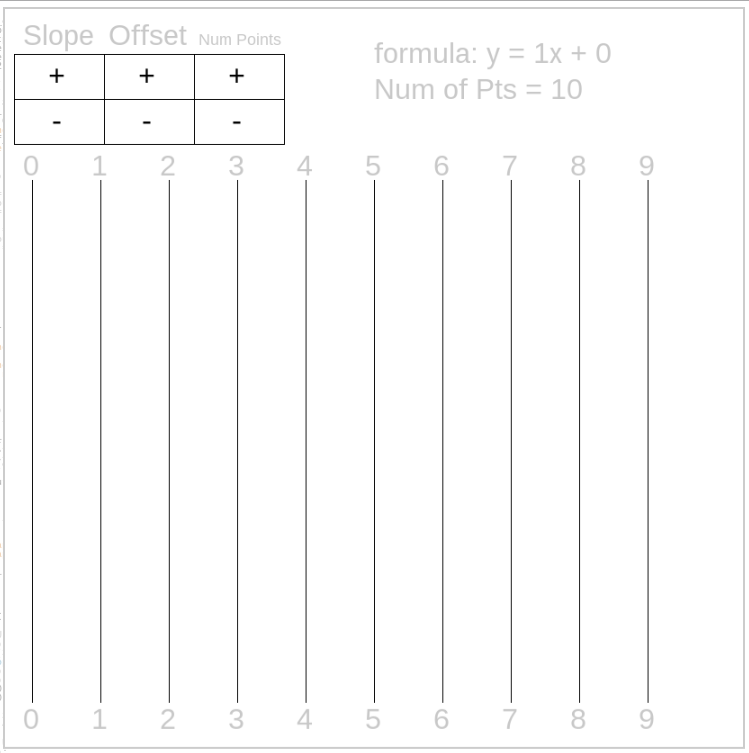So, this is just about the least interesting function, y = x (mod 10). So if x = 3, then y = 3 + 0 (mod 10) = 3. So draw a line from 3 to 3. Try the interactive yourself, click to change the function and see how it changes the picture.

Here’s another boring function: y = 0x + 0 (mod 10).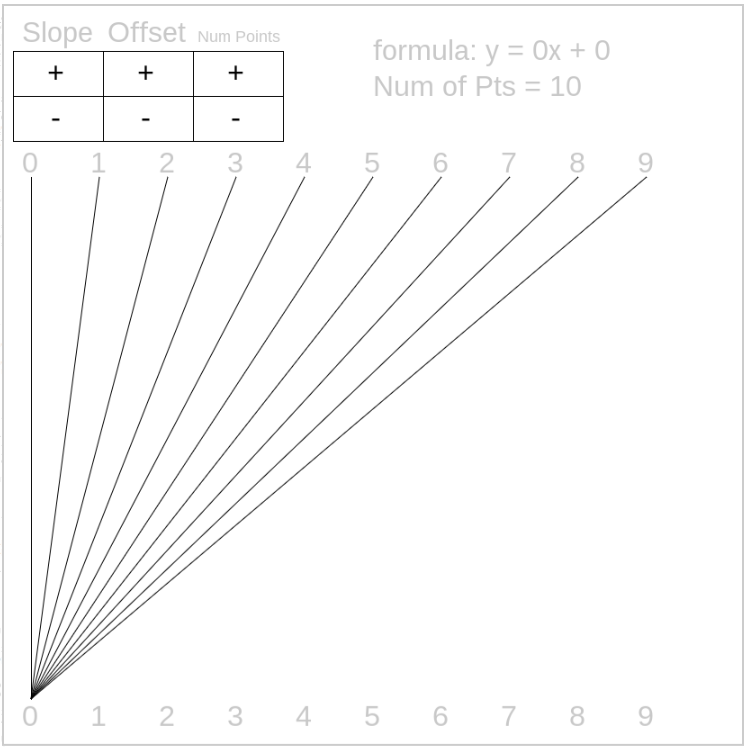Ok, lets get to the interesting stuff, y = 7x + 8 (mod 10). Take x = 4, so y = 28 + 8 (mod 10) = 36 (mod 10) = 6. Draw a line from 4 to 6.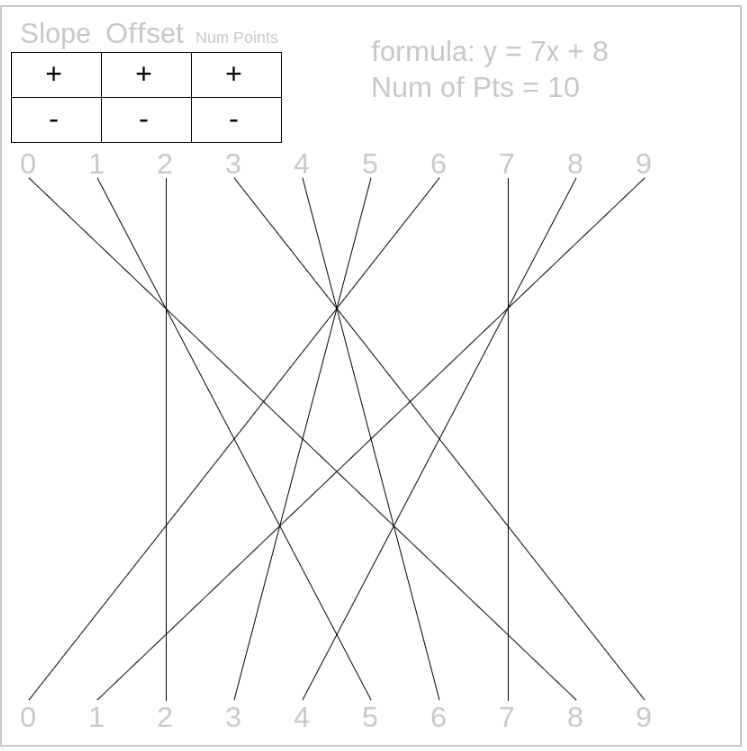Here’s another interesting picture: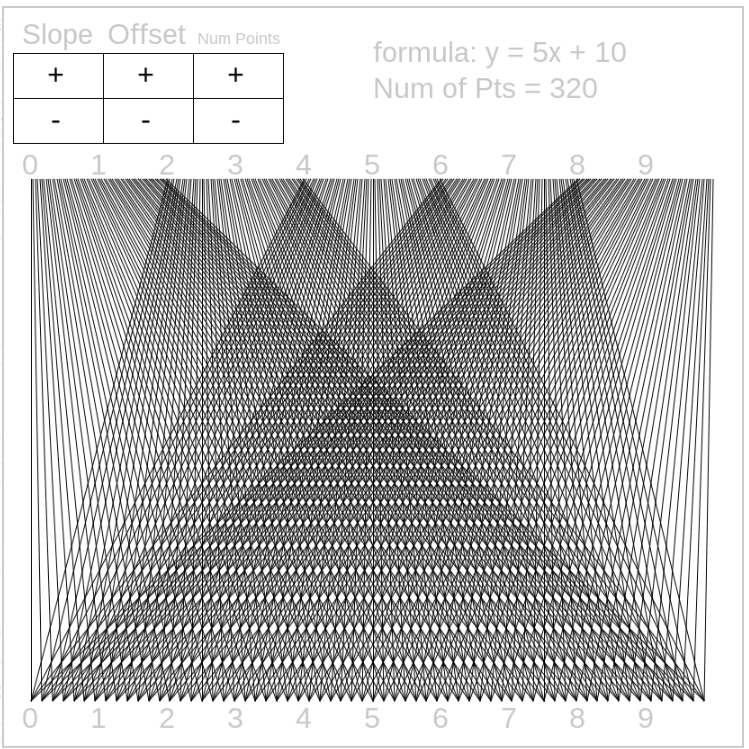If you really enjoy the picture that you’ve made then you can get a high definition version (5k by 5k) by copying the code, installing processing.org and clicking any key. The image will be in the same file as project. Very good for printing in large form if you’re lucky enough to have a big printer.

## Questions For You

How can I bring this into the math classroom?

Where does this fit?

Is modular arithmetic in anyone’s curriculum? If not, why not?

Should I (or you) put together a non-linear version? Exponential, powers, logarithmic?

Other thoughts?

Posted in Full Posts | Tagged , , | 4 Comments

## The Mandelbrot Set Viewed through Precalculus

I’ve given this presentation in November 2014 at AMTNYS in Syracuse, and in April 2015 at NCTM in Boston. I’ll be giving the presentation in May 2015 at the NY Master Teacher Conference, and in July 2015 at Twitter Math Camp in Claremont CA.

## Drive

Why use the Mandelbrot fractal to get the students to learn some Precalculus topics?

## How

Here are several good videos that can help you get started with the Mandelbrot set:

Next, you can walk through the lesson outline that I’ve made (pdf) and through the presentation that I gave for NCTM Boston (pdf).

Here are links to all the interactives:

Everything is also found here: bit.ly/mandelbrotfractal, including all the presentation material, the walkthrough, the interactives (and all the source code).

I had a ton of fun with this project and presentation, and I hope the students did too. Please let me know if you have any questions or comments. Thanks!

*Errors fixed thanks to a comment by J. Peter Matelski (one of the original Mandelbrot developers!)

Posted in Full Posts | | 5 Comments

## Using the arctan Power Series to Calculate Pi

(Note: this post is an extension on the calculating pi with python post from a couple of years back. Also here’s another way to inefficiently calculate pi with Buffon’s Needles.)

We’re currently working with Power series and Taylor series in Calculus.
One particularity pretty derivation is going from the series for$\frac{1}{(1-x)} = 1 + x + x^2 + x^3 ...$ to the series for$arctan(x) = 1 - \frac{x^3}{3} + \frac{x^5}{5} - \frac{x^7}{7} + ...$
Even better you can use this formula to calculate pi, since$arctan(1) = \frac{\pi}{4}$, so$\pi = 4 ( 1 - \frac{1}{3} + \frac{1}{5} - \frac{1}{7} + ...)$.
How quickly does this converge to pi? Let’s find out.
Here’s the first ten partial sums:
 n= 0 and the partial sum is 4.0 n= 1 and the partial sum is 2.666666666666667 n= 2 and the partial sum is 3.466666666666667 n= 3 and the partial sum is 2.8952380952380956 n= 4 and the partial sum is 3.3396825396825403 n= 5 and the partial sum is 2.9760461760461765 n= 6 and the partial sum is 3.2837384837384844 n= 7 and the partial sum is 3.017071817071818 n= 8 and the partial sum is 3.2523659347188767 n= 9 and the partial sum is 3.0418396189294032 n= 10 and the partial sum is 3.232315809405594 
Ok, that’s slow.
Here’s the first 100 terms:
 n= 0 and the partial sum is 4.0 n= 10 and the partial sum is 3.232315809405594 n= 20 and the partial sum is 3.189184782277596 n= 30 and the partial sum is 3.1738423371907505 n= 40 and the partial sum is 3.1659792728432157 n= 50 and the partial sum is 3.1611986129870506 n= 60 and the partial sum is 3.157984995168666 n= 70 and the partial sum is 3.155676462307475 n= 80 and the partial sum is 3.1539378622726155 n= 90 and the partial sum is 3.1525813328751204 n= 100 and the partial sum is 3.1514934010709914 
Still not to 3.14 even after adding 100 terms of this sequence?
Here’s the first 10,000 terms:
 n= 0 and the partial sum is 4.0 n= 1000 and the partial sum is 3.1425916543395442 n= 2000 and the partial sum is 3.1420924036835256 n= 3000 and the partial sum is 3.1419258758397897 n= 4000 and the partial sum is 3.1418425911015158 n= 5000 and the partial sum is 3.1417926135957908 n= 6000 and the partial sum is 3.1417592924821482 n= 7000 and the partial sum is 3.141735490326666 n= 8000 and the partial sum is 3.1417176379662446 n= 9000 and the partial sum is 3.1417037523562383 n= 10000 and the partial sum is 3.1416926435905346 
Two factors of ten more than the last set and still not to 5 digits of precision?
Lastly, here’s the first ten million approximations:
 n= 0 and the partial sum is 4.0 n= 1000000 and the partial sum is 3.1415936535887745 n= 2000000 and the partial sum is 3.1415931535894743 n= 3000000 and the partial sum is 3.1415929869229293 n= 4000000 and the partial sum is 3.1415929035895926 n= 5000000 and the partial sum is 3.1415928535897395 n= 6000000 and the partial sum is 3.141592820256488 n= 7000000 and the partial sum is 3.1415927964468655 n= 8000000 and the partial sum is 3.141592778589681 n= 9000000 and the partial sum is 3.141592764700862 n= 10000000 and the partial sum is 3.1415927535897814 

I really don’t want to calculate this by hand if after 10,000,000 additions, we only have 7 digits of precision.

(Question: why are all these even terms an over-estimate of pi?)

Graph of the first 1000 partial sums:Let’s compare that to Chudnovsky’s algorithm:
 Chudnovsky n= 1 3.1415926535897342076684535915782983407622332609156 n= 2 3.1415926535897932384626433835873506884758663459963 n= 3 3.1415926535897932384626433832795028841971676788547 n= 4 3.1415926535897932384626433832795028841971693993750 n= 5 3.1415926535897932384626433832795028841971693993750 n= 6 3.1415926535897932384626433832795028841971693993750 n= 7 3.1415926535897932384626433832795028841971693993750 

Wow, this algorithm maxed out the 51 digits of precision after 4 iterations. Crazy.

Here’s the python code for using arctan to approximate pi:

from decimal import *

#Sets decimal to 50 digits of precision
getcontext().prec = 50

#This program uses the power series for arctan to calculate pi
#arctan(x) = sum (n = 0 to infinity) (-1)^n * (x^(2n+1))/(2n+1)
#So to calculate pi, compute (arctan (1)) = pi/4 = 1 - 1/3 + 1/5 - 1/7 +...

quarterpi = Decimal(0)
n = 0

while True:
quarterpi = quarterpi + Decimal(-1)**n / (Decimal(2*n + 1))
#this line allows the code to print only every 10 lines, increase this number
#to speed up the calculations and reduce the printing
if (n % 10 == 0):
print((quarterpi*Decimal(4)))
n += 1



And here’s the chudnovsky python code:

from decimal import *

#Sets decimal to 50 digits of precision
getcontext().prec = 50

def factorial(n):
if n<1:
return 1
else:
return n * factorial(n-1)

def chudnovskyBig(n): #http://en.wikipedia.org/wiki/Chudnovsky_algorithm
pi = Decimal(0)
k = 0
while k < n:
pi += (Decimal(-1)**k)*(Decimal(factorial(6*k))/((factorial(k)**3)*(factorial(3*k)))* (13591409+545140134*k)/(640320**(3*k)))
k += 1
pi = pi * Decimal(10005).sqrt()/4270934400
pi = pi**(-1)
return pi
print ("Chudnovsky")
for i in range(1,50):
print ("n=",i," ", chudnovskyBig(i))

Here’s a sample of the stuff going up on that site.